Next: B. Analytical Fourier Transformations Up: PhD Thesis Heinrich Kirchauer Previous: Physical modeling.

# A. Approximation of the Inclination Factor

During the derivation of the Fresnel and the Fraunhofer diffraction formulae (4.14) and (4.15), respectively, the inclination factor occurring in (4.10) has been approximated under the assumption that the source xs as well as the observation point xo are located near the optical axis (cf. Figure 4.1). We will now explain how this approximation is obtained.

First we define three angles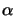,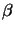, and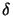guided by Figure 4.1 and shown in Figure A.1:(A.1)

The approximation of the inclination factor--compare (4.12) with (4.14) or (4.15)--thus writes to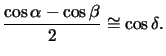(A.2)

Furthermore we introduce the two auxiliary angles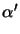and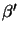as illustrated in Figure A.1. In the situation of interest the two points xs and xo are near the optical axis and thus,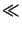1 yielding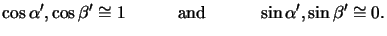(A.3)

By noting that the sum of the angles of the two triangles {xo,x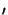,x} and {xs,x,x} equals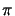, we geta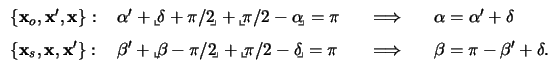(A.4)

With these two expressions forandand the approximations listed in (A.3), the derivation of (A.2) is straight forward: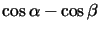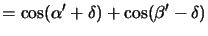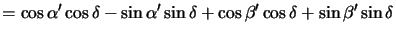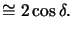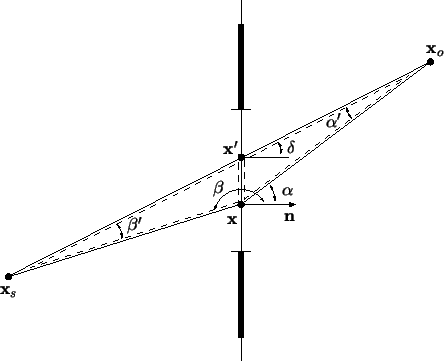#### Footnotes

... geta
The terms forming one common triangle-angle are indicated by delimiters (cf. Figure A.1).Next: B. Analytical Fourier Transformations Up: PhD Thesis Heinrich Kirchauer Previous: Physical modeling.
Heinrich Kirchauer, Institute for Microelectronics, TU Vienna
1998-04-17